﻿ SQL Between - TutorialsTrend
This Angular tutorial helps you get started with Angular quickly and effectively through many practical examples.
`

# SQL-Between Clause

In this tutorial, we will learn how to use BETWEEN operator to match values within a given range with different value types.

#### BETWEEN Operator

The BETWEEN operator is used to match values within a given range. The BETWEEN operator values can be numbers, text, or dates. we can use BETWEEN operator in the WHERE clause of INSERT, UPDATE, SELECT, and DELETE Query.

Syntax

``````SELECT column_name1, column_name2 FROM table_name
WHERE column_name || expression BETWEEN start_expression AND end_expression

or

SELECT column_name1, column_name2
FROM table_name
WHERE column_name BETWEEN start_expression AND end_expression``````

If a value in the column or the expression is matched within a given range, the result of the BETWEEN operator is TRUE.

For the demo purpose, we will use the below table. The table looks like that: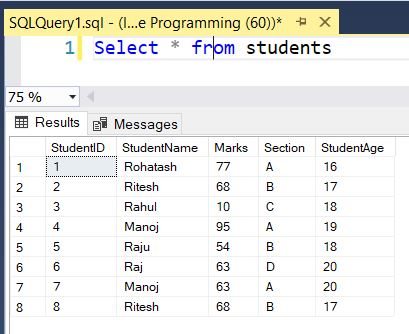#### BETWEEN Operator Examples

Let’s take some examples of using the BETWEEN operator to understand how it works.

SQL Server BETWEEN with Numbers Example

The following query finds the Students whose marks are between 63 and 90.

``````SELECT StudentID, StudentName, Marks, StudentAge
FROM students
WHERE Marks BETWEEN 63 AND 90``````

The result of the above statement is shown in the following output: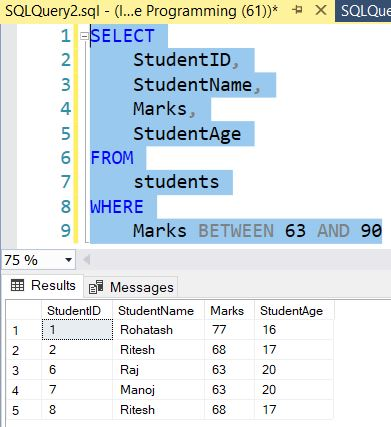SQL Server BETWEEN with Text Example

The following query finds the Students whose names are between M and R. Here, the SQL command selects all students where the item name lies between M and R.

``````SELECT StudentID, StudentName, Marks, StudentAge
FROM students
WHERE StudentName BETWEEN 'm' AND 'r'``````

The result of the above statement is shown in the following output: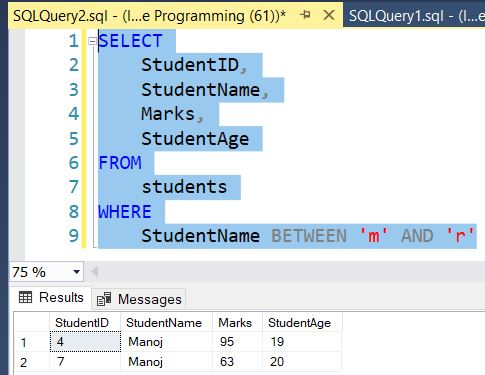SQL Server BETWEEN with Date Example

Now we add one column in the above table named createdate and define some dates.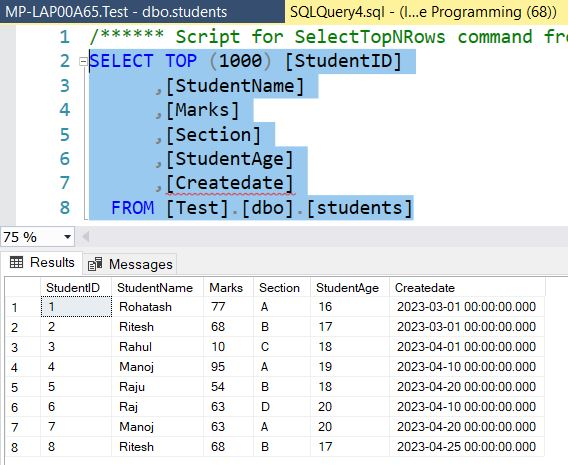The following query finds the Students whose createdate are between 2023-04-01 and 2023-04-20.

``````SELECT * FROM students
WHERE [Createdate] BETWEEN '2023-04-01' AND '2023-04-20';``````

The result of the above statement is shown in the following output: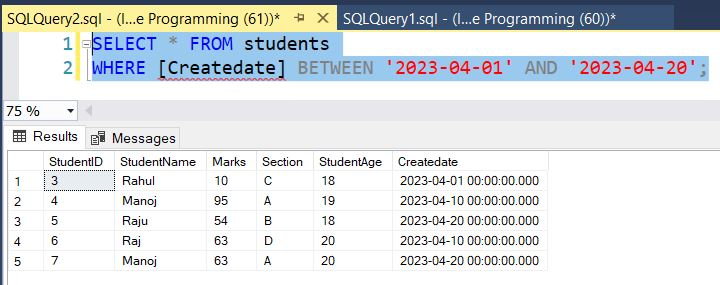SQL Server NOT BETWEEN Operator

As the name suggested it is just apposite the BETWEEN Operator. To negate the result of the BETWEEN operator, you use NOT BETWEEN operator as follows:

``````SELECT StudentID, StudentName, Marks, StudentAge
FROM students
WHERE Marks NOT BETWEEN 63 AND 90``````

The result of the above statement is shown in the following output: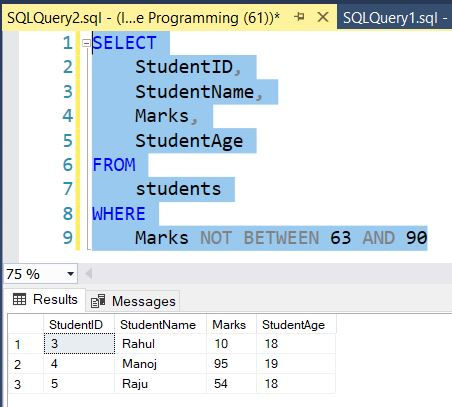Next